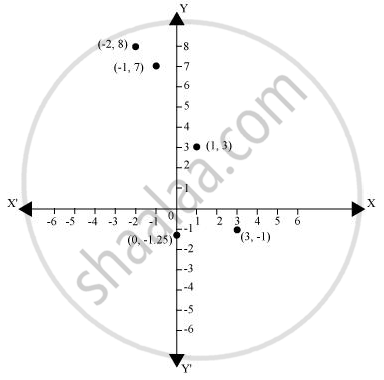# Plot the points (x, y) given in the following table on the plane, choosing suitable units of distance on the axes - Mathematics

Plot the points (x, y) given in the following table on the plane, choosing suitable units of distance on the axes.

 x -2 -1 0 1 3 y 8 7 -1.25 3 -1

#### Solution

The given points can be plotted on the Cartesian plane as follows.Concept: Plotting a Point in the Plane If Its Coordinates Are Given.
Is there an error in this question or solution?

#### APPEARS IN

NCERT Class 9 Maths
Chapter 3 Coordinate Geometry
Exercise 3.3 | Q 2 | Page 65